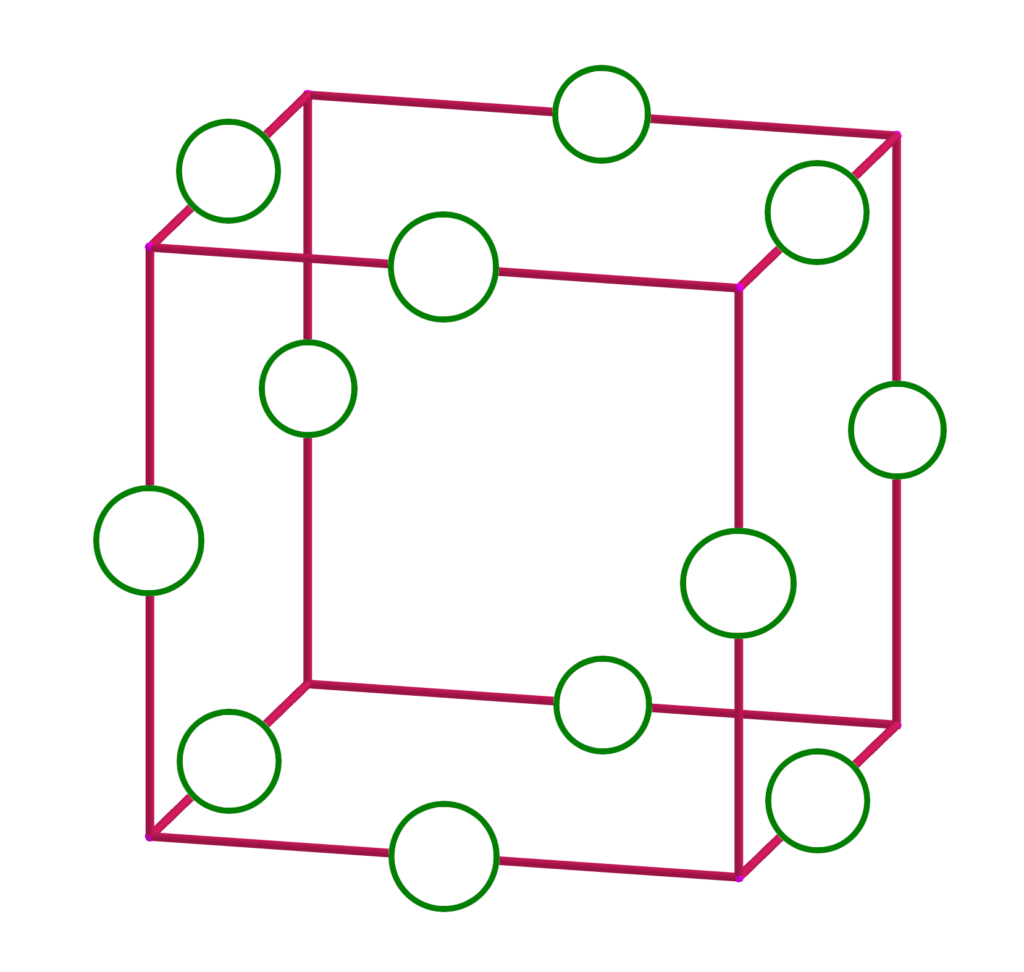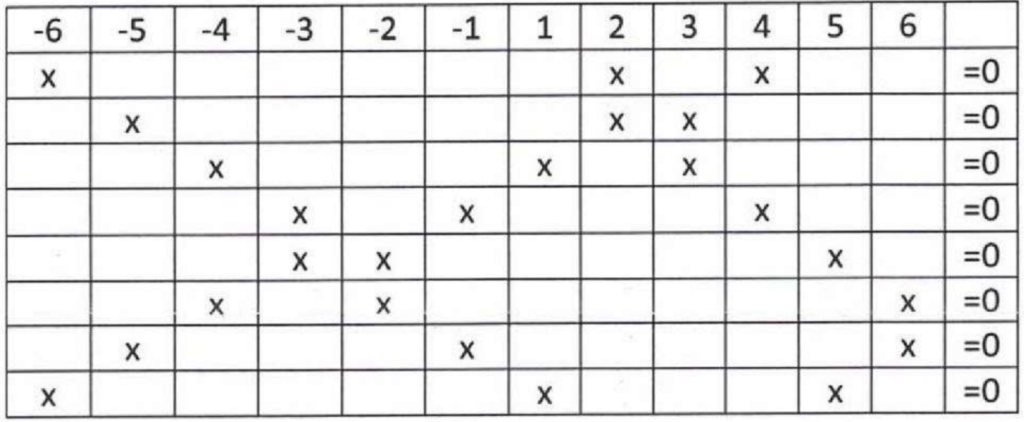# The Prisoner's Dilemma

### D3. Zero Sum Game

Contributed by Ian Stewart, University of WarwickFigure 1: Number all edges to make the sum of those meeting at each corner zero.

Is it possible to number the edges of a cube using each of the numbers -6, -5, -4, -3, -2, -1, 1, 2, 3, 4, 5, and 6 once, so that for every vertex, the sum of the numbers on the edges that meet there is zero? (See figure 1 for a diagram of the cube with a space on each edge to fill in — the labels on the three red lines that meet at any corner should add up to zero.) What about if instead you use the 12 consecutive integers from -5 to 6, inclusive?

This problem originally appeared in the Prisoner’s Dilemma in the 2022 Winter issue of the PMP Newsletter. Solutions are no longer being accepted for this Dilemma.

Show solution?
Solution.

The Prisoner’s Dilemma received a solution to the first part by reader Jesse Waite from Ft. Leavenworth, Kansas. Jesse realized that there are eight vertices in a cube, so that it’s necessary to find eight sums of three of the numbers equaling zero. In addition, each number is used exactly twice in the eight sums (once for each end of the edge). That led Jesse to create the table in figure 2 showing a very nice pattern of three entries in each row and two in each column, so that the sums across are all zero.Figure 2: Eight sums of three numbers using -6 to -1 and 1 to 6 twice each, all equal to 0.

Armed with this pattern, Jesse was able to fit the numbers onto the edges of the cube as shown in figure 3, solving the problem.